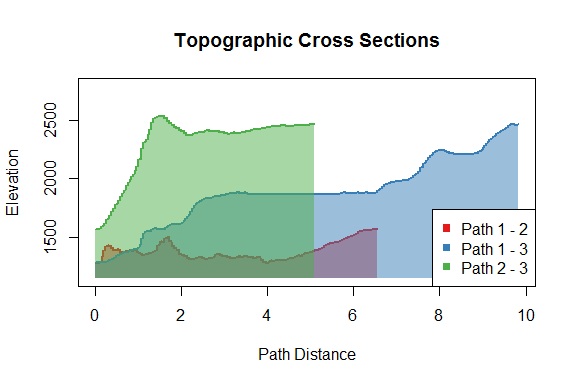Introduction to topoDistance

The topoDistance package provides functions for calculating topographic distances and identifying and plotting topographic paths. Unlike the topographically-corrected distances calculated by some GIS software, which just adjust for elevational changes along a straight-line path between points, topoDistance calculates the distance along the shortest topographic path between points, which is more likely to realistically reflect biological movement on a topographically complex landscape (Wang & Shaffer, 2017).

Identifying topographic paths and calculating topographic distances is valuable for a variety of disciplines, from landscape genetics to community ecology. For example, topographic distances have been shown to correlate strongly with genetic distances (e.g. Goldberg & Waits, 2010; Murphy et al., 2010; Wang & Shaffer, 2017) and with differences in community composition (e.g. Cañedo-Argüelles et al., 2015; Dong et al., 2016; Glassman, et al., 2017) in a variety of systems.

Calculating Topographic Distances

Identifying shortest topographic paths and calculating topographic distances is straightforward with the topoDist() function. All that is required is an elevation raster (DEM) and a set of geographic points. The topoDistance package contains a raster dataset that includes a DEM for part of Yosemite National Park. We can start by loading the data and plotting the DEM.

plot(Yosemite$DEM, col = terrain.colors(99))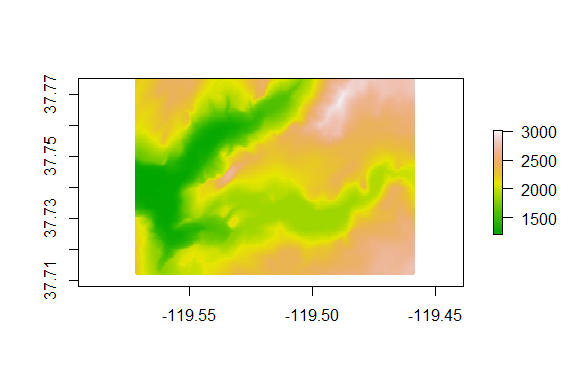Next we define the set of points between which we will find the topographic paths. The format for the points can be a two-column matrix (longitude and latitude) or a SpatialPoints object. xy <- matrix(ncol = 2, byrow = TRUE, c(-119.5566, 37.72474, -119.5157, 37.76688, -119.4718, 37.76078)) colnames(xy) <- c("longitude", "latitude") xy #> longitude latitude #> [1,] -119.5566 37.72474 #> [2,] -119.5157 37.76688 #> [3,] -119.4718 37.76078 Now, to calculate the topographic distances and paths, we just need to call the topoDist() function. The topoDist() function generates a topographic distance surface by calculating the topographic distance between cells as the hypotenuse of their horizontal and vertical distances. These distances are assignd to the weights of vertices between the nodes for each cell on a landscape graph. topoDist() then calls on functions in the gdistance (van Etten, 2015) and igraph (Csardi & Nepusz, 2016) packages. To calculate the shortest topographic distances, we only need to specify the DEM and spatial coordinates to use. tdist <- topoDist(Yosemite$DEM, xy, paths = TRUE)
tdist
#> []
#>          1        2        3
#> 1    0.000 6703.930 9499.491
#> 2 6703.930    0.000 4671.203
#> 3 9499.491 4671.203    0.000
#>
#> []
#> SpatialLines:
#> class       : SpatialLines
#> features    : 3
#> extent      : -119.5565, -119.4719, 37.72479, 37.76688  (xmin, xmax, ymin, ymax)
#> crs         : +proj=longlat +datum=WGS84 +ellps=WGS84 +towgs84=0,0,0
#> Coordinate Reference System (CRS) arguments: +proj=longlat
#> +datum=WGS84 +ellps=WGS84 +towgs84=0,0,0

When paths = TRUE, topoDist() returns a list that contains [] the matrix of pairwise topographic distances between points (in meters or projection units, depending on the coordinate reference system) and [] a SpatialLines object containing the shortest topographic paths. Whe paths = FALSE, topoDist() only returns the matrix of topographic distances.

Mapping Topographic Paths

We can map where the shortest topographic paths are on the landscape by using the topoPathMap() function.

topoPathMap(Yosemite$DEM, xy, topoPaths = tdist, type = "hillshade", pathWidth = 4, cex = 2, bg = "blue")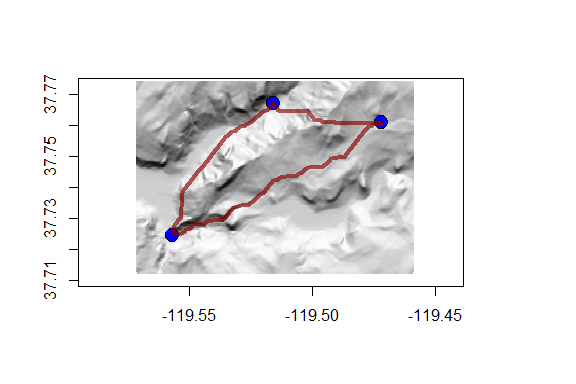Topographic Least Cost Paths The shortest topographic path distances calculated by the topoDist() function consider only the total overland distances between points, but in some cases we may also want to account for landscape resistance to movement. Resistance-based measures of geographic distance, like those produced by least cost path analysis (LCPA) and circuit theory analysis, are popular in landscape genetics and movement ecology (McRae & Beier, 2007; Wang & Shaffer, 2017). To combine resistance-based distance with topographic distance, the topoDistance package provides the topoLCP() function for calculating topographic least cost path distances. The topoLCP() function topographically corrects the resistance distances by multiplying the resistance along vertices in the landscape graph by the topographic distance between the nodes they connect The second layer in the Yosemite raster stack is a raster of habitat suitability values from a species distribution model (SDM) constructed for the western fence lizard (Sceloporus occidentalis) in the Yosemite National Park region. We can plot this layer with a common SDM color scheme to see how habitat suitability is distributed. sdmColors <- colorRampPalette(c("blue", "green", "yellow", "orange", "red"), space = "rgb", interpolate = "linear") plot(Yosemite$SDM, col = sdmColors(99))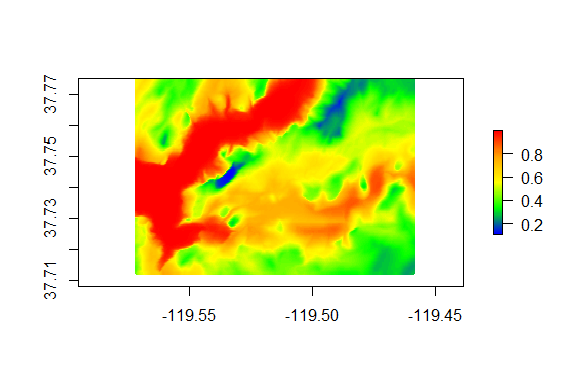More suitable habitat is presumed to have lower resistance to movement, so we can use this raster as a conductance surface (where conductance is the inverse of resistance). Along with the DEM and spatial coordinates, we can then use the topoLCP() function to identify topographic least cost paths and calculate their distances.

tLCP <- topoLCP(Yosemite$DEM, costSurface = Yosemite$SDM, pts = xy, paths = TRUE)
tLCP
#> []
#>          1        2         3
#> 1     0.00 6775.930 13567.722
#> 2  6775.93    0.000  9772.036
#> 3 13567.72 9772.036     0.000
#>
#> []
#> SpatialLines:
#> class       : SpatialLines
#> features    : 3
#> extent      : -119.5565, -119.4719, 37.72479, 37.76688  (xmin, xmax, ymin, ymax)
#> crs         : +proj=longlat +datum=WGS84 +ellps=WGS84 +towgs84=0,0,0
#> Coordinate Reference System (CRS) arguments: +proj=longlat
#> +datum=WGS84 +ellps=WGS84 +towgs84=0,0,0

As with topoDist(), topoLCP returns a list that contains [] the matrix of pairwise topographic distances between points and [] a SpatialLines object containing the shortest topographic paths when paths = TRUE. Whe paths = FALSE, topoLCP() returns only the matrix of topographic distances.

We can use the topoPathMap() function to map where the topographic least cost paths on the landscape. When a raster is supplied for the costSurface argument, the resistance surface will be superimposed on the elevation raster (if type = “hillshade”).

topoPathMap(Yosemite$DEM, xy, topoPaths = tLCP, type = "hillshade", costSurface = Yosemite$SDM, pathWidth = 4, pathColor = "purple")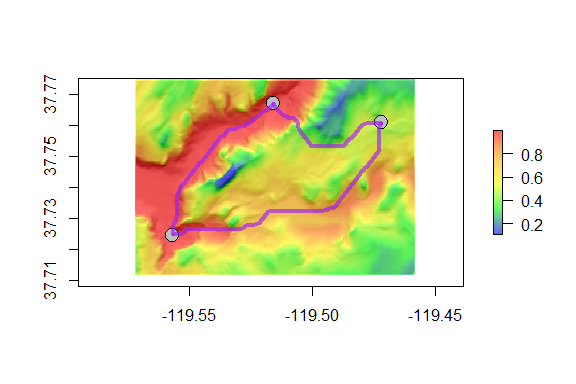We can always work with the topographic paths identified by topoDist() or topoLCP() using any functions that operate on SpatialLines objects. For example, if we want to compare the shortest topographic paths to the topographic least cost paths (TLCPs), we can plot the TLCPs using topoPathMap() and then plot the shortest topographic paths using the lines() function.

topoPathMap(Yosemite$DEM, xy, topoPaths = tLCP, type = "hillshade", costSurface = Yosemite$SDM, pathWidth = 4, pathColor = "purple")
lines(tdist[], lty = 2, lwd = 2)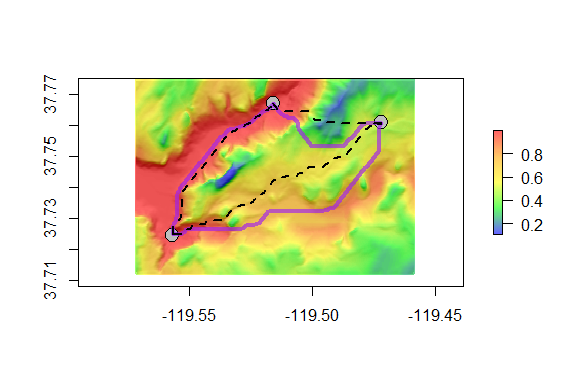Topographic Path Cross Sections

Finally, we can use the topoProfile() function to plot topographic cross sections (elevation profiles) for each of the paths. These can be useful for better understanding the trajectory of a path or identifying landscape features of interest along the paths. If we set the plot type to plotly (type = “plotly”) then topoProfile() generates an interactive plot that allows us to find the elevation and path distance for at any point along each path.

topoProfile(Yosemite\$DEM, topoPaths = tLCP, pts = 1000,
type = "base", singlePlot = TRUE)